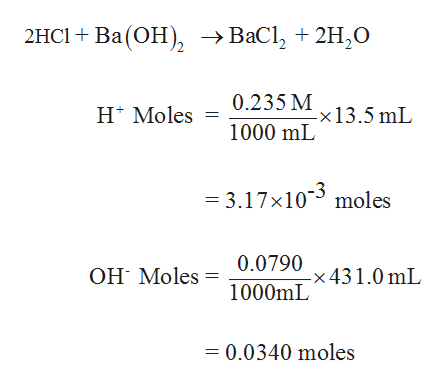# Hydrocholic acid (13.5 mL of 0.235 M) is added to 431.0 mL of 0.0790 M Ba(OH)2 solution. what is the concentration of the excess H+ or OH- ions left in this solution? assume that the volumes are additive.

Question
27 views

Hydrocholic acid (13.5 mL of 0.235 M) is added to 431.0 mL of 0.0790 M Ba(OH)2 solution. what is the concentration of the excess H+ or OH- ions left in this solution? assume that the volumes are additive.

check_circle

Step 1

The moles of HCl and the Ba(OH)2 moles are first calculated....help_outlineImage Transcriptionclose2HCI + Ba(ОН), —> ВаСl, + 2H,0 0.235 М 13.5 mL н Moles 1000 mL = 3.17x10 moles Он Moles 0.0790 х431.0 mL 1000 L 0.0340 moles fullscreen

### Want to see the full answer?

See Solution

#### Want to see this answer and more?

Solutions are written by subject experts who are available 24/7. Questions are typically answered within 1 hour.*

See Solution
*Response times may vary by subject and question.
Tagged in

### Inorganic Chemistry